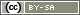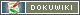# valueVar()

`float valueVar(string question)`

`float valueVar(string question, string|array items)`

`float valueVar(array variables)`

Calculates the uncorrected sample variance for the answer codes of all items in a question (e.g. a scale), for selected items, or for a list of variables.

• question – ID of a question (as a string)
• items – A list (string or array) of items
• variables – A list (array) of variable IDs as written in the Variables Overview

Note: This works in the same way as `valueSum()` – the parameters are described in this chapter in detail with various examples.